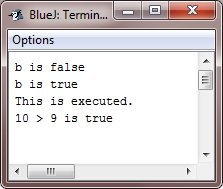# Java Boolean

Java has a primitive type, called boolean, for the logical values. It can have only one of the two possible values, true or false. This is the type returned by all the relational operators, as in the case of a<b.

boolean is also the type needed by conditional expressions that govern the control statements such as if and for.

## Boolean Example

Following is a program that illustrates the boolean type:

```/* Java Program Example - Demonstrate boolean values - Java Booleans */

public class JavaProgram
{
public static void main(String args[])
{

boolean b;

b = false;
System.out.println("b is " +b);
b = true;
System.out.println("b is " +b);

/* a boolean value can control the if statement */
if(b)
System.out.println("This is executed.");

b = false;
if(b)
System.out.println("This is not executed.");

/* outcome of a relational operator is a boolean value */
System.out.println("10 > 9 is " +(10>9));

}
}```

When the above Java program is compile and executed, it will produce the following output:There are three interesting things to notice about the above program. First, as you can see, when a boolean value is output by the method println(), "true" or "false" is displayed. Second, the value of a boolean variable is sufficient, by itself, to control the if statement. There is no requirement to write an if statement like this :
if(b == true) ...

Third, the outcome of relational operator, such as <, is a boolean value. This is why the expression 10>9 displays the value "true". And, the extra set of parentheses around 10>9 is necessary because the + operator has a higher precedence than >.

Tools
Calculator